Lecture 17
Simple Regression

Prediction
When I discussed correlation, I noted that a perfect correlation between two variables produces a line when plotted in a bivariate scatterplot.In this figure, every increase of the value of X is associated with an increase in Y without any exceptions. If we wanted to predict values of Y based on a certain value of X, we would have no problem in doing so with this figure. A value of 2 for X should be associated with a value of 10 on the Y variable, as indicated by this graph. Many times in scientific studies, we are interested in predicting values of Y based on X. For example, we may be interested in the blood cholesterol level of patients based on their daily intake of fat. We would be predicting values of cholesterol based on how much fat is in the diet. In the above picture, X is a perfect predictor of Y. We know exactly what the value of Y will be for a given value of X, because they all fall on a perfect line.

Error of Prediction--the "Unexplained Variance"
Usually, prediction won't be so perfect. Most often, not all the points will fall perfectly on the line. There will be some error in the prediction. For each value of X, we know the approximate value of Y but not the exact value.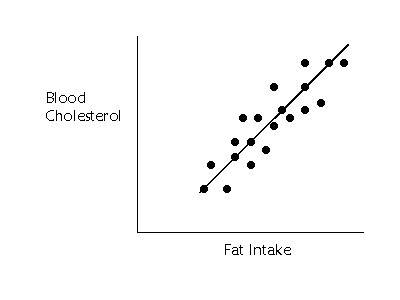In the above figure, blood cholesterol and fat intake are related, but we are not able to predict cholesterol levels perfectly based on the fat intake. The points in the graph that fall off of the line are not perfectly predicted. We can look at how much each point falls off the line by drawing a little line straight from the point to the line as shown below (sorry, my little lines are not perfectly aligned with the points).If we wanted to summarize how much error in prediction we had overall, we could sum up the distances (or deviations) represented by all those little lines. The middle line is called the regression line. Summing up the deviations of the points gives us an overall idea of how much error in prediction there is. Unfortunately, this method does not work very well. If we choose a line that goes exactly through the middle of the points, about half of the points that fall off of the line should be below the line and about half should be above. Some of the deviations will be negative and some will be positive, and, thus the sum of all of them will equal 0. Remember that when we added all the deviations of the scores from the mean, we also got 0? So, we pick a similar solution to the problem here. If we want to summarize the overall error in prediction, we sum up all the squared deviations from the regression line.

To calculate the deviations from the line we need a little notation. In regression analyses, we label the points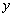. We try to predict the scores on the Y variable, given certain values of the X variable. So, we are primarily concerned with thescores. The (imaginary) scores that fall exactly on the regression line are called the predicted scores, and there is a predicted score for each value of X. The predicted scores are represented by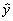(sometimes referred to as "y-hat", because of the little hat; or as "y-predict"). So the sum of the squared deviations from the predicted scores is represented byin which each y scores is subtracted from the predicted score (or the line) and then squared. Then all the squared deviations are summed up. Notice that this is a type of variation. It is the unexplained variation in the prediction of y when x is used to predict the y scores. Some books refer to this as the "sum of squares residual" because it is a measure of the residual variation (like a "residue" left over). Whatever we decide to call it, sum of the squared deviations from the regression line (or the predicted points) is a summary of the error of prediction.

The Regression Line and Ordinary Least Squares Criterion
Conversely, if we want to draw a line that is perfectly through the middle of the points, we would choose a line that had the squared deviations from the line. Actually, we would use the smallest squared deviations. This criterion for best line is called the "Least Squares" criterion or Ordinary Least Squares (OLS).

We use the least squares criterion to pick the regression line. The regression line is sometimes called the "line of best fit" because it is the line that fits best when drawn through the points. It is a line that minimizes the distance of the actual scores from the predicted scores.

Sum of Squares Regression--the Explained Variance
When we studied correlation, we saw that a linear relationship between two variables could be seen as a stream of points when plotted. When the correlation between x and y equals zero (no tendency for y to be large (or small) when x is large), there is just a group of random points. The graphs below show an example of each.On the left, there is no relationship between fat intake and cholesterol. On the right, there is a relationship. The regression line is flat when there is no ability to predict whatsoever. The regression line is sloped at an angle when there is a relationship.

The extent to which the regression line is sloped, however, represents the degree to which we are able to predict the y scores with the x scores. When I discussed the mean, I said the mean offered a way to summarize a group of scores. The scores are most likely to be close to the mean, because it is the middle. Therefore, if you wanted to guess at any one of the scores, the best guess would be the mean. When there is no relationship between x and y, the values of x are of no help in predicting the y scores, so we might as well use the mean of y, or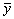to predict y scores. In the left hand side of the above figure, there is a flat line drawn at the mean. The best way to predict the y scores is with the mean of y. To the extent that there is a relationship between x and y, there will be some slope in the line of prediction. So, the degree to which the regression line is sloped compared to the mean, represents the amount we can predict y scores.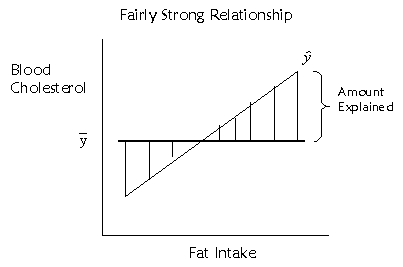The extent to which the regression line is sloped represents the amount we can predict y scores based on x scores, and the extent to which the regression line is beneficial in predicting y scores over and above the mean of the y scores. To represent this, we could look at how much the predicted points (which fall on the regression line) deviate from the mean. This deviation is represented by the little vertical lines I've drawn in the above figure. If we want to quantify this distance, we could use a similar method as before. The squared deviations of the predicted scores from the mean score, orrepresent the amount of variance explained in the y scores by the x scores.

Total Variation
We may also want to know about the total variation in the y scores. That is pretty easy to represent, because we have done the same thing elsewhere. The total variation is measured simply by the sum of the squared deviations of the y scores from the mean.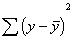It turns out that the explained sum of squares and the unexplained sum of squares add up to equal the total sum of squares. The variation of the scores is either explained by x or not.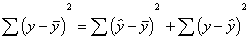Total sum of squares = explained sum of squares + unexplained sum of squares.

The Regression Equation
The regression equation is simply a mathematical equation for a line. It is the equation that describes the regression line. In algebra, we represent the equation for a line with something like this: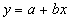a is the intercept, or the point at which the line travels through the y-axis (sometimes called the y-intercept), and b is the slope of the line. One can think of the y-intercept as the value of y when x is equal to 0. With a grid, we could find the slope of the line by counting how many points we have to go up to meet the line again after we have gone over one point to the right (remember "rise over run"). So the slope is a ratio of the increase in y with every point increase in x. With regression analysis, we need to find out what the equation of the line is for the best fitting line. What is the slope and intercept for the regression line? If the slope is zero, there is no relationship between x and y. If the slope is larger than 0 (or smaller, if the relationship is negative), there is a relationship.

To figure out the equation for the regression line, we first want figure out the slope, b. Here is the formula for that: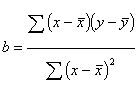Pretty simple, we've done similar formulas before. Notice that on the top of the formula, we compute the deviations of the x's from the mean of x and the deviation of the y's from the mean and multiple them. We do not square them. This top part of the equation can be called the covariance of x and y. The slope then represents the amount that x and y covary together relative to the overall variation of x. And sometimes the equation for b is written as: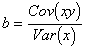the intercept, a, can then be obtained using b: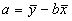where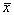andare the means of x and y respectively. In regression analysis, we are attempting to predict y based on x scores, so we represent the regression equation with asymbol to indicate a predicted score:I used the example of exam scores and time on examine in the correlation lecture to compute the regression equation. I'm not going to go through the steps here, because you should be getting the hang of this stuff. You might want to check my work as an exercise. If we solve the above equations for b and a, we would then wind up with an equation that looked like this: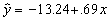meaning that the y-intercept was a value of -13.24 on the y axis, and the slope of the line is .69. The slope represents the amount of increase in y scores with one unit change in x scores. As we increase the time on the exam by 1 minute (x), we expect scores on the exam (y) to increase by .69. The y-intercept is not always meaningful. For instance, here, it means that when there are 0 minutes spent on the exam, the score on the test is about -13.